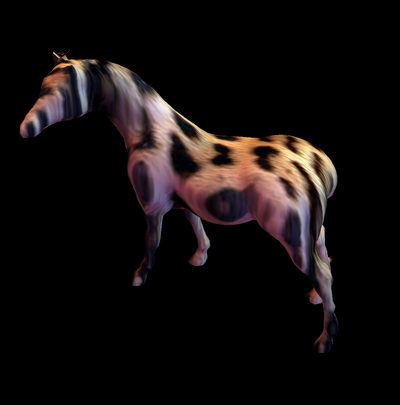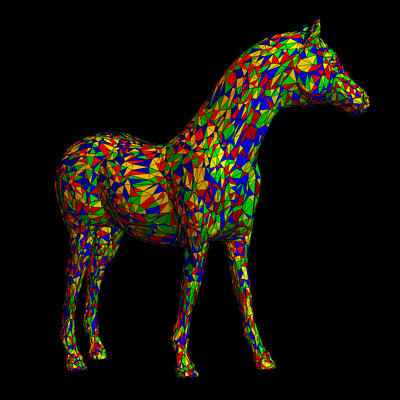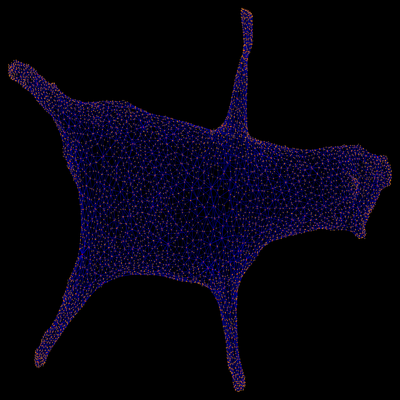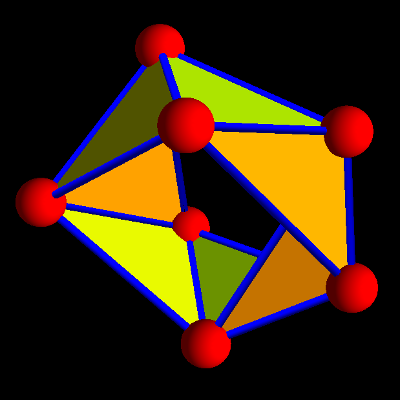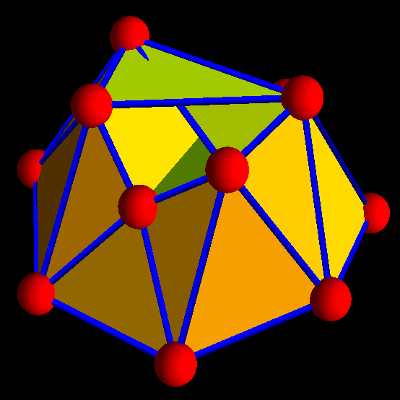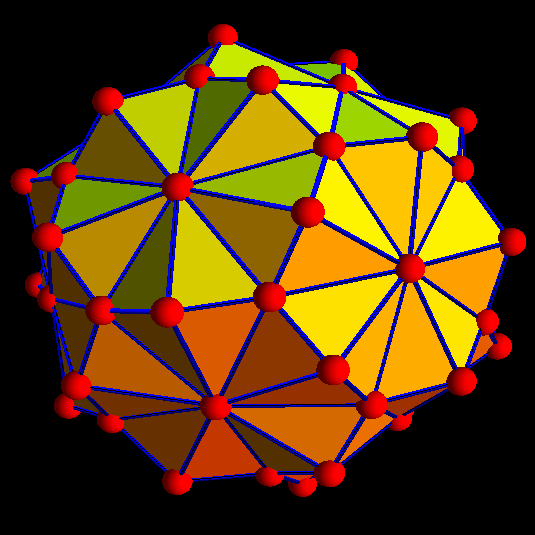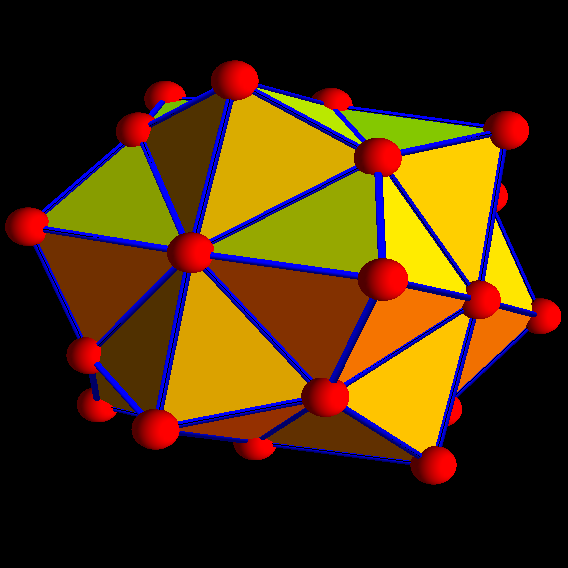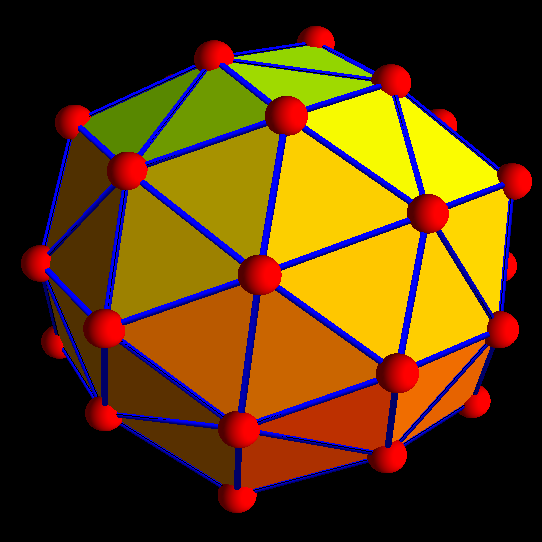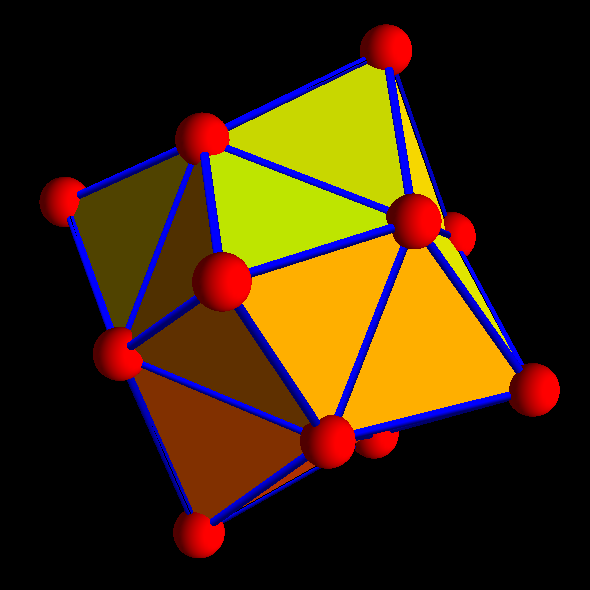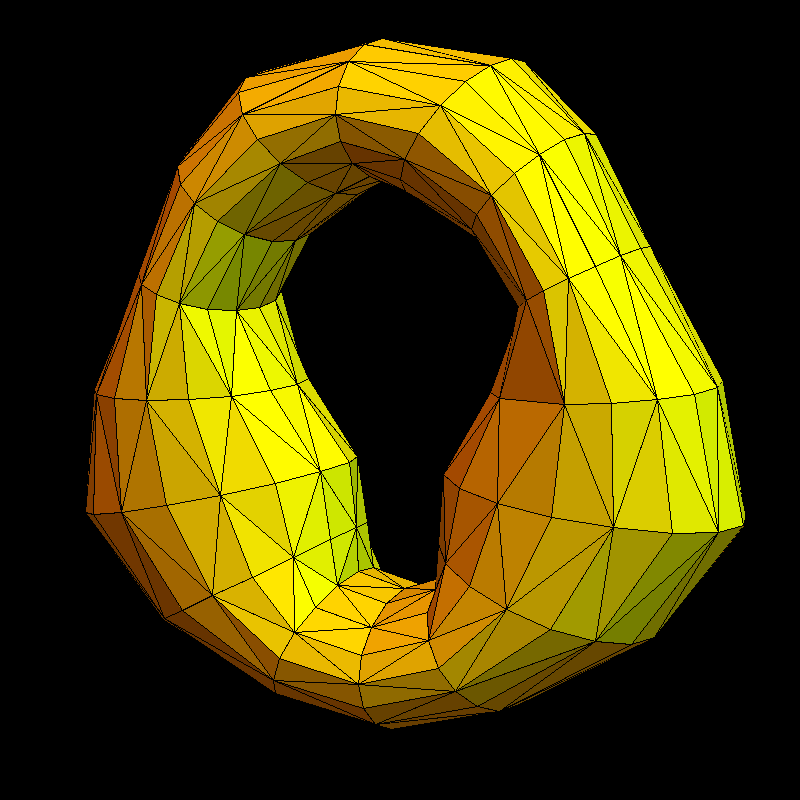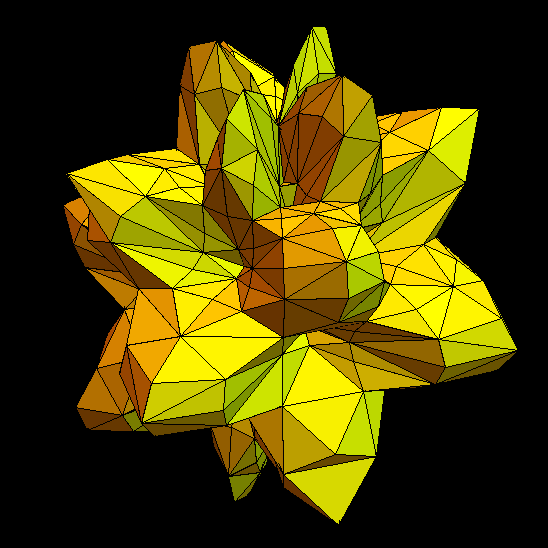# Geometric Graphs

This is a HCRP project in geometric graph theory with Jenny Nitishinskaya. This project took place from June 10 to August 7, 2014. This page is no more updated except for links to off-spring results. And more is still to come. For more news on graph geometry, see the main project page. Here are updates: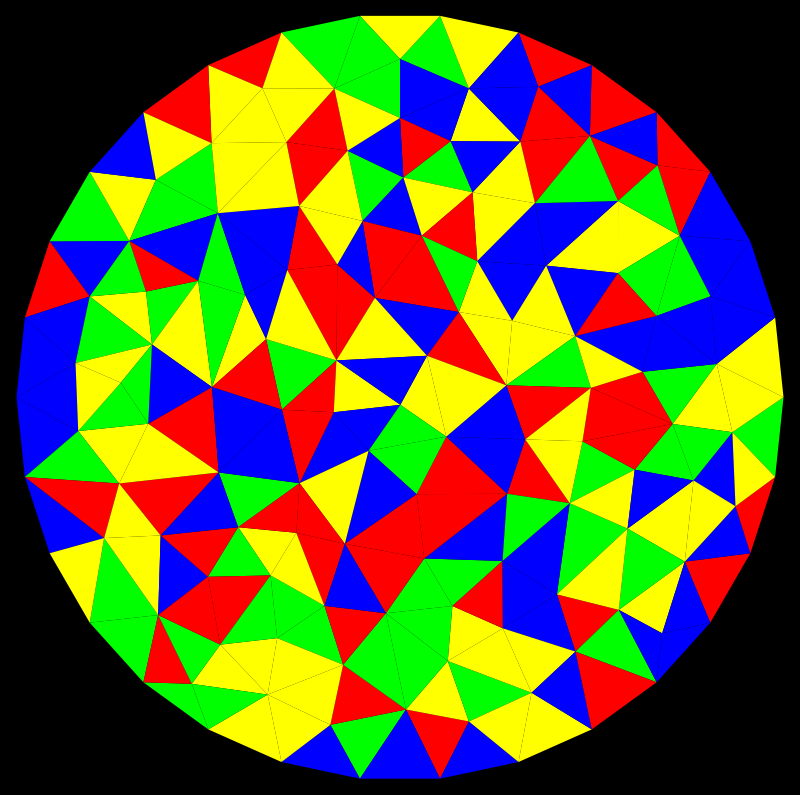Here are notes from June 13 to July 23, 2014, a research diary and not definitive. (All errors (and there are likely still many, are Oliver's)). Geometric two-dimensional graphs without boundary are graphs for which every unit sphere is a cyclic graph. Geometric two dimensional graphs with boundary are graphs for which every unit sphere is either a cyclic graph or an interval graph. For a geometric graph with boundary, the boundary components are a disjoint set of cyclic graphs. In this project, we aim to prove the following 4 color conjecture:

 HCRP 2014 conjecture: Any orientable geometric graph is 4 colorable. An orientable geometric graph with boundary is colorable with 4 colors so that each boundary component gets 3 colors only.

Note that this is not the famous 4 color problem but in the sphere or cylinder case we deal with a subclass. There are maps on the two torus which need 7 colors (but they are not geometric) and there are many geometric graphs (any boundary less graph of genus g larger than 0) which are not planar. In the proposal, we originally had suggested a stronger statement, not assuming orientability, but in the first meeting already, Jenny found a discretisation of the projective plane which has the chromatic number 5.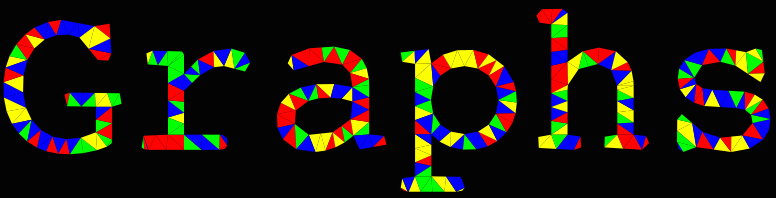But as the classical theorem, we use discrete differential geometry like Gauss-Bonnet and reduction arguments to get to the result. Unlike in the case of planar graphs, the differential geometric case is cleaner. And it is also new. The question of coloring geometric graphs has not appeared yet. The curvature in the interior is 1-|S(x)|/6 while the curvature at the boundary is (1/2-|S(x)|/3). The sum of the curvatures is the Euler characteristic. The basic strategy is to show that points with positive curvature can be reduced. Unlike the classical theorem, which needs case analysis so heavily that it is done only with the help of computers so far, we can avoid case analysis completely and hope even to get a constructive result in the sense that given an orientable geometric graph, we can build the coloring up. The later looks tough as coloring questions are always global. There is no "cellular automata" type construction which does the job. Enlarging a graph a bit can completely alter the coloring and closing a circular hole with a Moebius strip (picture left down) producing an unorientable graph even can change the chromatic number.